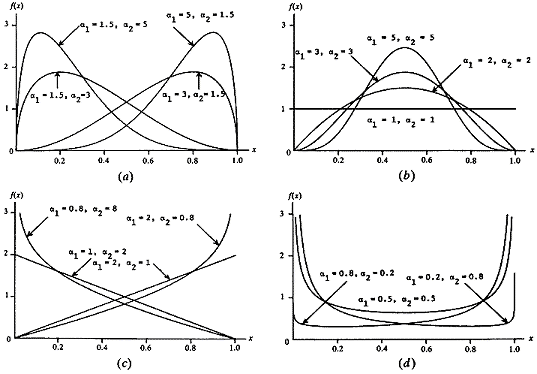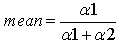Expert Modelling: Hands-on help from Rocscience Engineers. Register Here

# Beta Distribution

The Beta distribution is a very versatile function which can be used to model probability density curves of several different shapes, such as those shown below.

Beta (a1, a2) density functions (Law and Kelton, 1991)The form of the Beta distribution is determined by the shape parameters a1 and a2. Both a1 and a2 are always positive (greater than 0). The relationship between the shape parameters of the Beta distribution and the Slide input data is as follows:The standard deviation is the positive square root of the variance.

Note that the above equations apply to a beta random variable on [0,1]. To rescale and relocate to obtain a beta random variable on [a, b] of the same shape, use the transformation a + (b–a)X